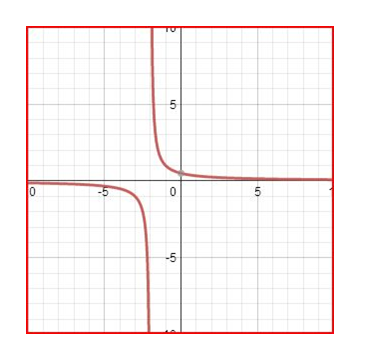Ch.1: Pre-Calc (Part 1)WorksheetSee all chapters
 Ch.1: Pre-Calc (Part 1) 3hrs & 29mins 0% complete WorksheetDownload the video lesson worksheet Ch.2: Pre-Calc (Part 2) 2hrs & 53mins 0% complete WorksheetDownload the video lesson worksheet Ch.3: Pre-Calc (Part 3) 1hr & 40mins 0% complete WorksheetDownload the video lesson worksheet Ch.4: Limits (Part 1) 2hrs & 25mins 0% complete WorksheetDownload the video lesson worksheet Ch.5: Limits (Part 2) 1hr & 53mins 0% complete WorksheetDownload the video lesson worksheet Ch.6: Derivatives (Part 1) 3hrs & 13mins 0% complete WorksheetDownload the video lesson worksheet Ch.7: Derivatives (Part 2) 2hrs & 26mins 0% complete WorksheetDownload the video lesson worksheet Ch.8: Applications of Derivatives (Part 1) 2hrs & 51mins 0% complete WorksheetDownload the video lesson worksheet Ch.9: Applications of Derivatives (Part 2) 2hrs & 1min 0% complete WorksheetDownload the video lesson worksheet Ch.10: Applications of Derivatives (Part 3) 3hrs 0% complete WorksheetDownload the video lesson worksheet Ch.11: Integrals 3hrs & 8mins 0% complete WorksheetDownload the video lesson worksheet

# Domain & Range

See all sections
Sections
Representation of a Function
Word Problems as Functions
Types of Functions
Asymptotes
Rational Functions
Computing Domain
Domain & Range

Concept #1: Domain and Range

Practice: Given the following set of coordinates, find the domain: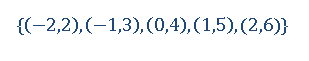Practice: Given the following set of coordinates, find the range: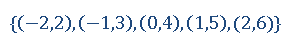Practice: Find the domain of the following function: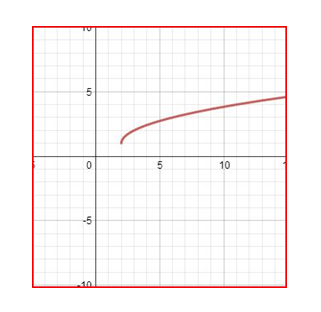Practice: Find the range of the following function: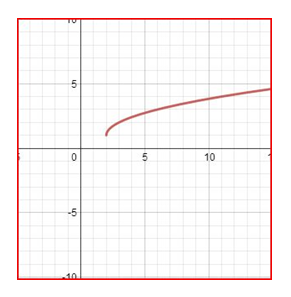Practice: Find the domain of the following function: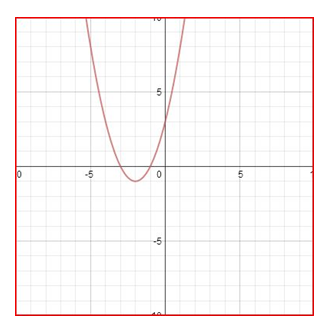Practice: Find the range of the following function:Practice: Find the domain of the following function,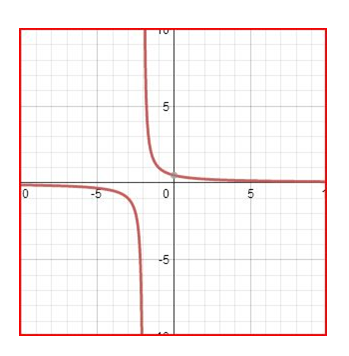Practice: Find the range of the following function: